Cosine

•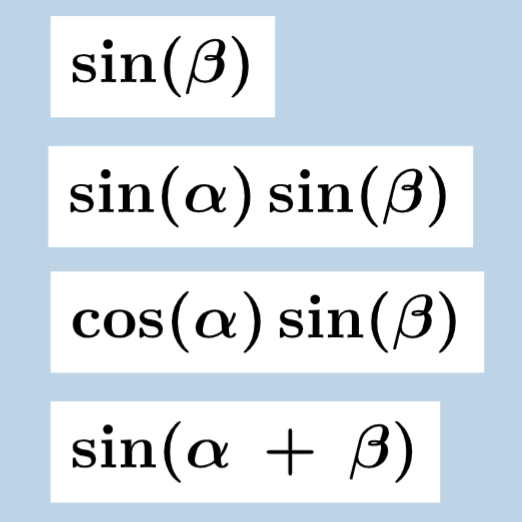Sine & Cosine of a Sum: Discovery

Activity

Tim Brzezinski

•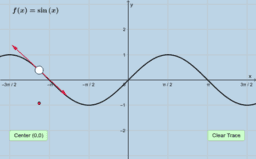Derivative of Sine & Cosine Functions (Quick Investigation)

Activity

Tim Brzezinski

•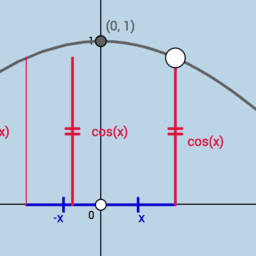Cosine Identity

Activity

Tim Brzezinski

•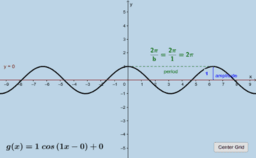Graphing Sine & Cosine Functions (II)

Activity

Tim Brzezinski

•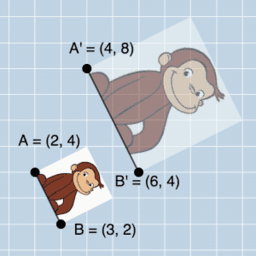Similarity & Right Triangle Trigonometry

Book

Tim Brzezinski

•Identifying Trig Ratios: Quick Formative Assessment

Activity

Tim Brzezinski

•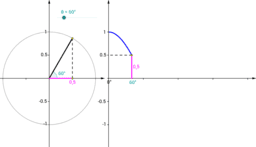Unit Circle and Cosine Graph

Activity

Anthony OR 柯志明

•Trigonometric Ratios (Right Triangle Context)

Activity

Tim Brzezinski

•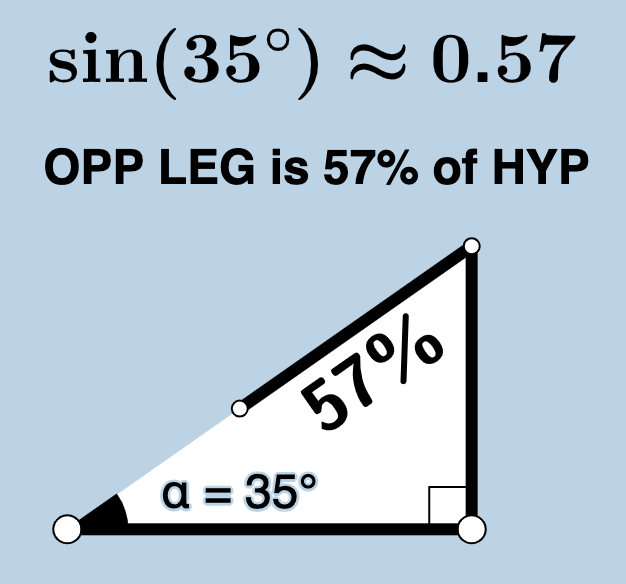Conceptual Illustrations: Trigonometric Ratios within Right Triangles

Activity

Tim Brzezinski

•Law of Cosines: Discovery

Activity

Tim Brzezinski

•What "CO" in COsine Means

Activity

Tim Brzezinski

•Unit Circle and the Trigonometric Functions

Activity

ayoob

•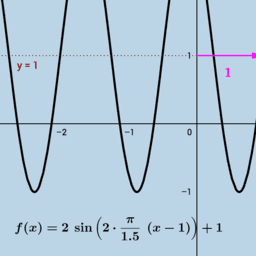Graphing Sine & Cosine Functions (I)

Activity

Tim Brzezinski

•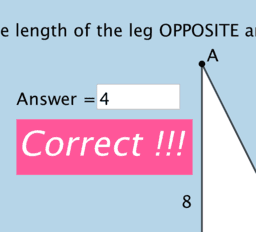Right Triangles: Identifying Sides WRT Acute Angles

Activity

Tim Brzezinski

•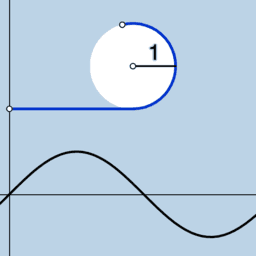Sine & Cosine Period Action (1)!

Activity

Tim Brzezinski

•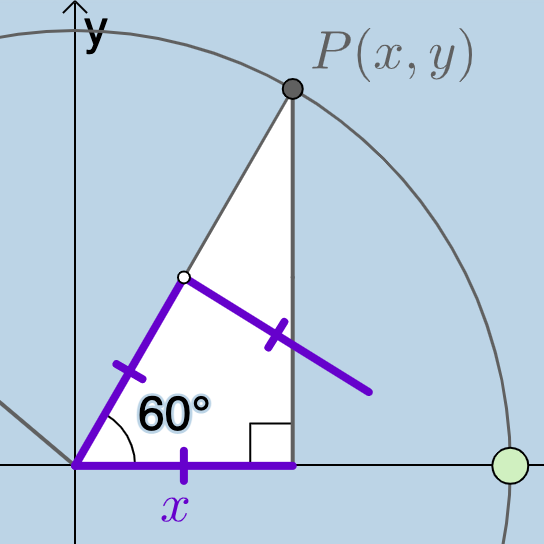Trig Function Values (60 Degrees)

Activity

Tim Brzezinski

•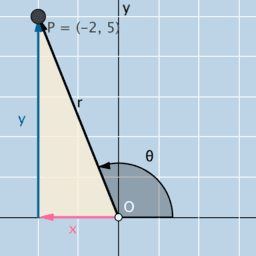Evaluating Trig Functions Given a Point on the Terminal Ray

Activity

Tim Brzezinski

•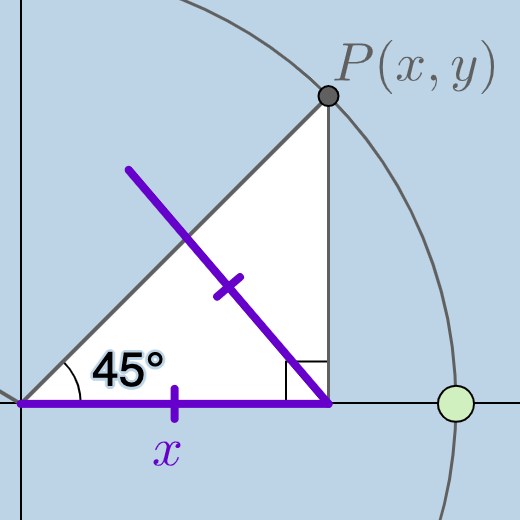Trig Function Values (45 Degrees)

Activity

Tim Brzezinski

•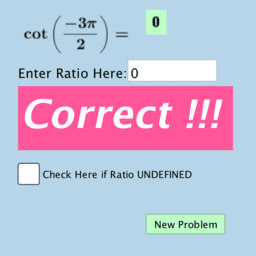Quiz 1: Trigonometric Functions of Special Angles

Activity

Tim Brzezinski

•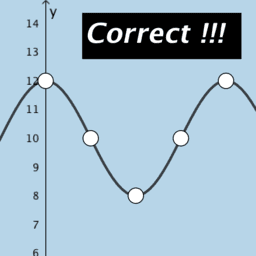Quiz: Graphing Sine & Cosine Functions (Amplitude & Vertical Shift Only)

Activity

Tim Brzezinski

•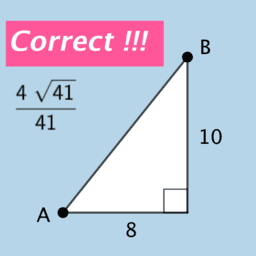Finding Exact Trig Ratios: Quiz Question Generator

Activity

Tim Brzezinski

•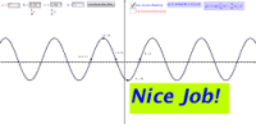Sine & Cosine Function Modeling: Finding Correct Parameters

Activity

Tim Brzezinski

•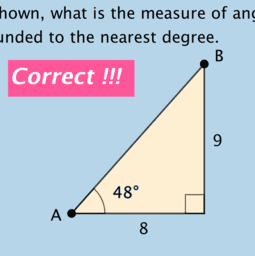Finding Acute Angles of Right Triangles

Activity

Tim Brzezinski

•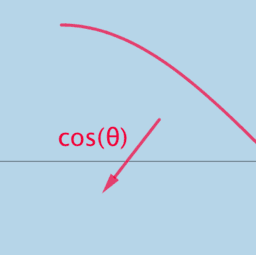Unit Circle to Sine and Cosine Functions

Activity

Tim Brzezinski